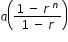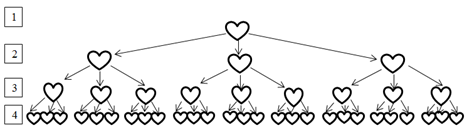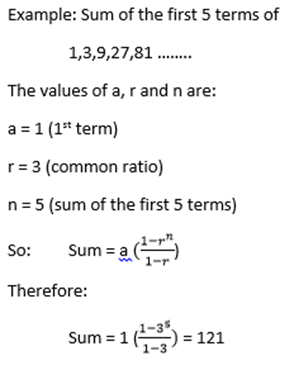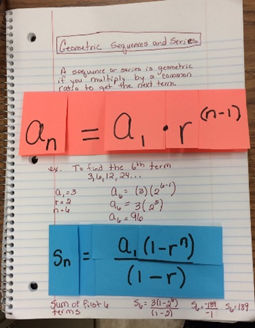# Access Point #: MAFS.912.A-SSE.2.AP.4aArchived Access PointExport Print
Use the formula for the sum of finite geometric series to solve problems.Clarifications:

#### Essential Understandings

Concrete:

• List the terms for a geometric series using a table or a graphic organizer.
(For example: 1, 3, 9, 27…)• Use manipulatives to find the sum of the terms of a geometric series.
(For example: The sum of the 4 terms is 40.)• Identify the first term of a geometric series.
• Identify the common ratio of a geometric series.
• Identify the desired number of terms for the summation.
Representation:
• Understand the following concepts and vocabulary: a partial sum of an infinite series, finite, geometric series, common ratio, terms, initial value, summation, arithmetic series, exponent.
• Determine the values to substitute into the formula for the sum of a finite geometric series.• Use a tool to calculate the sum of a finite geometric series (e.g., calculator, Click Here)
• Substitute values into the formula for the sum of a finite geometric series.General Information
Number: MAFS.912.A-SSE.2.AP.4a
Category: Access Points
Cluster: Write expressions in equivalent forms to solve problems. (Algebra 1 - Supporting Cluster) (Algebra 2 - Major Cluster)

Clusters should not be sorted from Major to Supporting and then taught in that order. To do so would strip the coherence of the mathematical ideas and miss the opportunity to enhance the major work of the grade with the supporting clusters.

## Related Standards

This access point is an alternate version of the following benchmark(s).

## Related Courses

This access point is part of these courses.
1200310: Algebra 1
1200320: Algebra 1 Honors
1200330: Algebra 2
1200340: Algebra 2 Honors
1200380: Algebra 1-B
1200400: Foundational Skills in Mathematics 9-12
1207310: Liberal Arts Mathematics
1200410: Mathematics for College Success
1200700: Mathematics for College Algebra
7912060: Access Informal Geometry
7912070: Access Mathematics for Liberal Arts
7912080: Access Algebra 1A
7912090: Access Algebra 1B
1200315: Algebra 1 for Credit Recovery
1200335: Algebra 2 for Credit Recovery
1200385: Algebra 1-B for Credit Recovery
7912065: Access Geometry
7912075: Access Algebra 1
7912095: Access Algebra 2
1200387: Mathematics for Data and Financial Literacy

## Related Resources

Vetted resources educators can use to teach the concepts and skills in this access point.

## Student Resources

Vetted resources students can use to learn the concepts and skills in this access point.

## Parent Resources

Vetted resources caregivers can use to help students learn the concepts and skills in this access point.